# Horizontal Asymptotes (3 Key Ideas & How To Find Them)

When graphing functions, it’s always useful to avail ourselves of different tools to help with the process. Asymptotes are a huge help when graphing!

While asymptotes are not part of the graph, they serve as guidelines to estimate function values. Likely, you are already familiar with vertical asymptotes but today’s discussion will focus on horizontal asymptotes.

## What is a horizontal asymptote?

Horizontal asymptotes are horizontal lines that help us determine the end behavior of a graph.

Consider the rational function p(x) = (4x3 – 3x2 + 10) / (x3 – x2 + x), pictured below.

Notice, as the x-values get bigger and bigger in the positive direction, the function values (the y-coordinates) approach the dotted line y = 4. Similarly, as the x-values get bigger and bigger in the negative direction, the function values approach the line  y = 4 .

The horizontal line y=4 is called a horizontal asymptote of the function.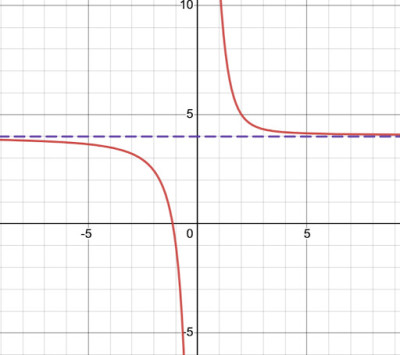Here is the graph of the rational function p(x) = (4x3 – 3x2 + 10) / (x3 – x2 + x), with a horizontal asymptote at y = 4.

A horizontal asymptote is a good approximation for how the function is behaving for very large positive or negative inputs. The function values approach the horizontal asymptote for large positive values and large negative values of x.

### What kinds of functions have horizontal asymptotes?

There are many types of functions that have horizontal asymptotes. In a high school math class, the most common functions that could have horizontal asymptotes are rational functions

A rational function will have one horizontal asymptote or no horizontal asymptotes. It depends on the degree of the numerator and denominator. Let’s look at rational functions in greater detail.

### Horizontal asymptotes of rational functions

When determining the horizontal asymptote of a rational function, we only need to consider the highest degree term in the numerator and denominator. For large input values, the other terms will be insignificant. Recall that the degree of a polynomial in one variable is the highest power of the terms in the polynomial.

For rational functions, there are 3 cases we need to consider in order to determine whether or not we have a horizontal asymptote.

#### Case 1

Degree of the numerator < Degree of the denominator

Let’s consider a specific example to see if we can then make a generalization about this case.

• f(x) = (2x2 – 10x + 3) / (x3 – 2)

To determine the horizontal asymptote, we’ll take the limit as x→∞ and as x→-∞ .

The only terms in the numerator and denominator that will matter are the highest power terms. So we can “cancel” out other terms that won’t be significant when x is very large.

Hence, the horizontal asymptote for f(x) is y = 0.

Let’s glance at Desmos to confirm this result: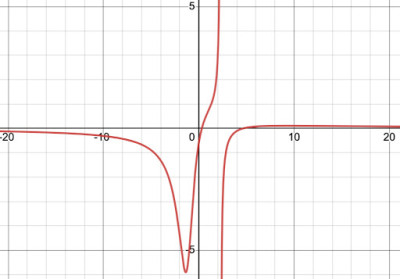Here is the graph of the rational function f(x) = (2x2 – 10x + 3) / (x3 – 2), with a horizontal asymptote at y = 0.

Indeed, the x-axis, which is the equation y = 0, is the horizontal asymptote for f(x). When the input values are small, the horizontal asymptote is not a good approximation for the function values.

In this case, if the degree of the numerator is less than the degree of the denominator, we will always get a horizontal asymptote at the line y=0.

#### Case 2

Degree of the numerator > Degree of the denominator

Let’s look at a specific example:

• g(x) = (6x2 – 13x + 5) / (x – 2)

Does this function have a horizontal asymptote? If so, what is it?

To answer the question, we need to think about the limits as  x→∞ and as x→-∞. Notice, the degree of the numerator is 2 and the degree of the denominator is 1.

If we imagine plugging in a really large x value, say 1,000,000, then hopefully it’s evident that the numerator will grow much faster than the denominator because the numerator has a higher degree.

If we compare all of the terms in both the numerator and denominator, the term 6x2 will grow much faster than the other terms. This means that the numerator will be much larger than the denominator, so the graph will increase without bound.

There is no horizontal asymptote. Let’s verify this by evaluating the limits:

As x→∞, 6x increases without bound so there is no limit and thus no horizontal asymptote. Similarly,

As  x→-∞, -6x decreases without bound so there is no horizontal asymptote. A quick look at Desmos confirms this result: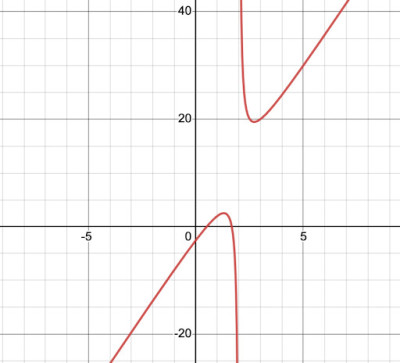Here is the graph of the rational function g(x) = (6x2 – 13x + 5) / (x – 2), with no horizontal asymptotes.

Notice, there isn’t a horizontal line that can approximate the end behavior of this function. Anytime the degree of the numerator is greater than the degree of the denominator, the function won’t have any horizontal asymptotes.

#### Case 3

Degree of the numerator = Degree of the denominator

Let’s consider one more case:

• h(x) = (3x2 + 2x + 1) / (x2 – x + 3)

What, if any, is the equation of the horizontal asymptote?

As before, we only need to consider the highest degree terms in the numerator and denominator. The other terms won’t matter for very large input values.

To determine the horizontal asymptote, we’ll take the limit as x→∞ and as x→-∞ .

Hence, the horizontal asymptote is y = 3. This is the ratio of the leading coefficients!

The leading coefficient of the numerator is 3 and the leading coefficient of the denominator is 1. So the horizontal asymptote is y=3/1=3.

A quick look at Desmos confirms this result.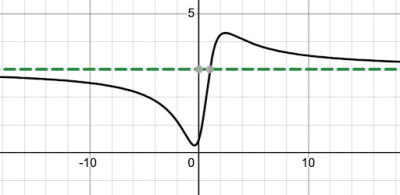Here is the graph of the rational function h(x) = (3x2 + 2x + 1) / (x2 – x + 3), with a horizontal asymptote at y = 3.

When the x-values are small, the horizontal asymptote is not a good approximation for the function. In this example, the function crosses the horizontal asymptote near x = 0.

This can happen with horizontal asymptotes, but not with vertical asymptotes.

Another point of interest: as x→∞, the function values approach the line y = 3 from above the asymptote. But, as x→-∞, the function values approach the horizontal asymptote from below.

### What other types of functions have horizontal asymptotes?

#### Logistics Function

Logistics functions are functions that describe population growth where the growth is limited by the environment.

Here is an example of a logistics function with two horizontal asymptotes:

• y=21+0.1x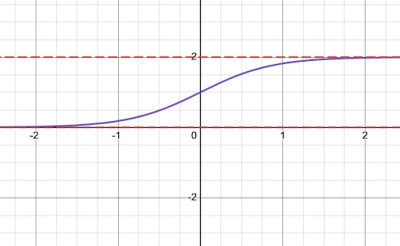The graph of the logistic function f(x) = 21 + 0.1x, with horizontal asymptotes at y = 2 and y = 0 (the x-axis).

Note: the horizontal asymptotes are y=2 and the x-axis y=0.

#### Inverse Tangent Function

The function f(x) =arctan(x) also has two horizontal asymptotes, as seen below.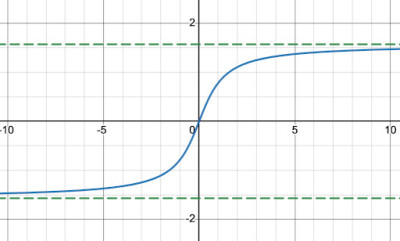The graph of the function f(x) = arctan(x), with horizontal asymptotes at y = π/2 and one at y = -π/2.

Notice that the inverse tangent function has two horizontal asymptotes, one at y=π/2 and one at y=-π/2.

### What is a slant asymptote?

Let’s go back to Case 2, where the degree of the numerator is greater than the degree of the denominator. There is a special situation that arises if the numerator has degree that is 1 greater than the degree of the denominator.

The function won’t have a horizontal asymptote in this case, as we already observed. But, it will have a slant asymptote. A slant asymptote is a non-vertical/non-horizontal line that approximates the values of the function for large positive inputs and large negative inputs.

#### Example

Consider the function f(x) = (x2 + x – 6) / (x – 1). Find the slant asymptote.

Solution

First, we can see that the degree of the numerator is 2 and the degree of the denominator is 1 so we will have a slant asymptote. To find the slant asymptote, we need to use long division.

The quotient will give us the equation for the slant asymptote.

We can use this polynomial long division calculator to divide the polynomials.

As we can see, the quotient is x+2 with a remainder of -4. For slant asymptotes, we’re not interested in the remainder, only the quotient so the equation  y=x+2 is the slant asymptote for the function.

Let’s confirm this result on Desmos.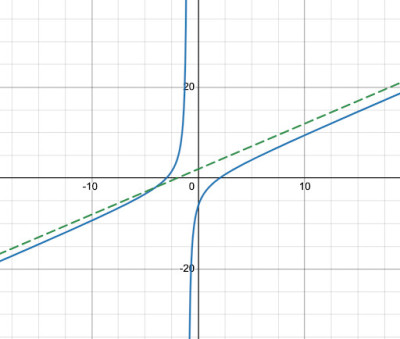The graph of the function f(x) = (x2 + x – 6) / (x – 1), which has a slant asymptote of y = x + 2.

Sure enough, the green dotted line is the slant asymptote! The function values will approach the slant asymptote for very large positive or negative values of x.

It turns out, we can also have asymptotes that aren’t lines! For a rational function, if the degree of the numerator is 2 more than the degree of the denominator, then a parabola will serve as an oblique asymptote! That’s a topic for another day.

Remember, horizontal asymptotes are super helpful when graphing functions. An asymptote acts as a guideline for sketching a function.

Typically, before graphing a function, we should draw in the asymptotes. And remember, horizontal asymptotes aren’t subject to the same restrictions as vertical asymptotes. A function can cross a horizontal asymptote, which makes them pretty special!